time constant

Also found in: Dictionary, Thesaurus, Medical, Acronyms, Wikipedia.

Time constant

A characteristic time that governs the approach of an exponential function to a steady-state value. When a physical quantity is varying as a decreasing exponential function of time as in Eq. (1), or as an increasing exponential function as in Eq. (2), the approach to the steady-state value achieved after a long time is governed by a characteristic time T as given in Eq. (3). This time T is called the time constant. (1)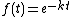(2)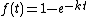(3)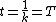When time t is zero, f(t) in Eq. (1) has the magnitude 1, and when t equals T the magnirude is 1/e. Here e is the transcendental number whose value is approximately 2.71828, and the change in magnitude is 1 - (1/e) = 0.63212. The function has moved 63.2% of the way to its final value. The same factor also holds for Eq. (2).

The initial rate of change of both the increasing and decreasing functions is equal to the maximum amplitude of the function divided by the time constant.

The concept of time constant is useful when evaluating the presence of transient phenomena.

McGraw-Hill Concise Encyclopedia of Physics. © 2002 by The McGraw-Hill Companies, Inc.

time constant

Symbol: τ. A measure of the speed with which an electronic system can respond to a sudden change at its input.
Collins Dictionary of Astronomy © Market House Books Ltd, 2006
The following article is from The Great Soviet Encyclopedia (1979). It might be outdated or ideologically biased.

Time Constant

a generalized parameter that describes the dynamic properties (time lag) of a given entity and that has the dimension of time. Any complex physical process can be represented as a set of simpler processes, each of which can be mathematically described by a first- or second-order linear differential equation. These simple processes are called basic components in the theory of automatic control. For example, a first-order aperiodic basic component is described by the equation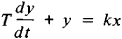where x is the input coordinate, y the output coordinate, k a proportionality factor, and T the time constant.

Time constants are widely used to calculate the dynamics of various entities or processes under study. Thus, the heating of a substance within a closed container at constant ambient temperature is described by the equation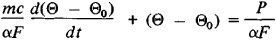where m is the mass and c the heat capacity of the substance, a is the coefficient of heat transfer into the medium surrounding the container, F is the reduced cooling surface, Θ is the ambient temperature, Θ0 is the initial temperature of the substance, and p is the heat energy suddenly imparted to the substance from a heater at the initial moment of time t = 0. The change in the temperature of the substance is determined by the equation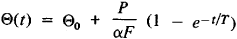where T = mc/ α F is the time constant. The greater the time constant, the less rapidly the heating proceeds.

The time constant of transient phenomena in electric circuits characterizes the rate at which current or voltage varies in the circuit. For example, when a capacitor with capacitance C is charged from a DC source with electromotive force E. through a resistance r that is much greater than the internal resistance of the current source, the voltage across the capacitor plates varies according to the law

uc = E(1 - e-1/T)

where T = rC is the time constant that determines the rate at which the charging process occurs. The time constant of an electric circuit with inductance L is L/r.

A. V. KOCHEROV

time constant

[′tīm ‚kän·stənt]
(physics)
The time required for a physical quantity to rise from zero to 1-1/ e (that is, 63.2%) of its final steady value when it varies with time t as 1 -e -kt .
The time required for a physical quantity to fall to 1/ e (that is, 36.8%) of its initial value when it varies with time t as e -kt .
Generally, the time required for an instrument to indicate a given percentage of the final reading resulting from an input signal. Also known as lag coefficient.
McGraw-Hill Dictionary of Scientific & Technical Terms, 6E, Copyright © 2003 by The McGraw-Hill Companies, Inc.
References in periodicals archive ?
After depression, synaptic responses recover with a time constant of seconds.
However, again from the Bode diagram in its phase angle format, the presence of three time constants is observed.
In the single frequency cases it can be noted that the ionospheric contribution is significantly smaller for the shorter smoothing time constant due to the smoothing time dependent term (18).
where [F.sub.oi] - transfer function of the current control loop; [F.sub.Ri] - transfer function of the current controller; [K.sub.FC] - transfer constant of the frequency converter; [K.sub.I] - transfer constant of the current sensor; [K.sub.AD] - transfer constant of the A/D converter; [L.sub.S] - stator inductance; [R.sub.S] - stator resistance; [T.sub.i] - time constant of the current control loop (CL); [T.sub.NCi] - uncompensated time constant of the current CL; [T.sub.Si] - sampling period of the current CL; [T.sub.S] - stator time constant; [T.sub.FC] - time constant of the frequency converter; [sigma] - total leakage constant.
It is interesting to see how the time constant varies depending on the operating conditions of the heat pump.
If the filter-based current sensing technique is used for over current protection, and time constant L [tau] is greater than time constant [[[tau].sub.C], the over current protection circuit can trip at a lower than desirable current.
The fast process associated with the time constant [[tau].sub.1] was on the order of 103 s and was influenced by the coating thickness and porosity.
This model is particularly intuitive, because it illustrates how more capacitance becomes available as the time constant of the charging cycle is increased.

Site: Follow: Share:
Open / Close